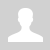Python. Strings. Functions and methods

11.2.2.3 apply functions and string processing methods

Python. Strings. Functions and methods

String is a indexed sequence of characters.

 Function/Method Example Description s1 + s2 s1 += s2 s1 = "Hello " s2 = "world" s = s1 + s2  print(s) # "Hello world" Concatenation of strings means all characters from s1 come first, and then all characters from s2 s * n s *= n s1 = "Ra" s = s1 * 4  print(s)  # "RaRaRaRa" String s repeated n times s1 in s2 s1 = "own" s2 = "brown" print(s1 in s2) # True print("ron" in s2) # False Checking that substring s1 is contained in s2 s1 not in s2 s1 = "own" s2 = "brown" print(s1 not in s2) # False print("ron" not in s2) # True Checking that substring s1 is not contained in string s2 The same as not (s1 in s2) len(s) s = "school" print(len(s))  # 6 String length s[i] 'school' == 'h' 'school'[-1] == 'l' s = "star" print(s) # s print(s[-3]) # t i-th element of the string, negative i - for counting from the end s[start:stop:step] s = 'school' print(s[:2]) # 'sc' print(s[2:5]) # 'hoo' print(s[::2]) # 'sho' Slice a string s.count(s2, a1, an) s = "abracadabra" print(s.count("a"))  # 5 print(s.count("a", 2))  # 4 print(s.count("a", 2, 9))  # 3 s2 = "bra" print(s.count(s2))  # 2 count() method returns the number of occurrences of the substring in the given string. s1.find(s2)  s1.rfind(s2) s = 'abracadabra' print(s.find('bra'))  # 1 print(s.rfind('bra'))  # 8 print(s.find('bar'))  # -1 The index of the beginning of the first or last occurrence of the substring s2 in s1 (will return -1 if s2 not in s1) s.startswith(s2)  s.endswith(s2) print('school'.startswith('sc')) # True print('school'.endswith('ml')) # False Checking if s starts with s2 or ends with s2 s.isdigit()    s.isalpha()   s.isalnum() print('123'.isdigit())  # True print('12A'.isdigit())  # False print('abc'.isalpha()) # True print('ab3'.isalpha()) # False print('E315'.isalnum()) #True print('E3 15'.isalnum()) #False Checking that all characters in string s are numbers,   letters (including Cyrillic),   numbers or letters s.islower()  s.isupper() print('hi!'.islower()) # True print("Uralsk".islower()) # False print('PY123'.isupper()) # True print('NuR%2'.isupper()) # False Checking that the string s does not contain large letters,   small letters. Note that for both of these functions, punctuation marks and numbers give True s.lower()  s.upper() print('Welcome!'.lower()) # welcome! print('Welcome!'.upper()) # WELCOME! The string s, in which all letters are converted to lower or upper case, are replaced with lower or upper case. s.capitalize() s.title() print('welcome back'.capitalize()) # Welcome back print('welcome back'.capitalize()) # Welcome Back String s, in which the first letter is uppercase String s, in which all words will be capitalized. s.lstrip()  s.rstrip()  s.strip() print('  Welcome!  '.lstrip()) # 'Welcome!  ' print('  Welcome!  '.rstrip()) # '  Welcome!' print('  Welcome!  '.strip()) # 'Welcome!' String s with whitespace characters (spaces, tabs) removed from the beginning, end, or both sides s.ljust(k, c)   s.rjust(k, c) print('Comment'.ljust(10, '.'))   # Comment... print('Comment'.rjust(10, '.'))   # ...Comment Adds the required amount to the left or right characters c so that the length s reaches k s.join(a) print('  '.join(['Computer', 'science'])) # Computer science Concatenates (joins) strings from list a through s s.split(s2) s = "Computer science" print(s.split()) # ["Computer", "science"] print(s.split('e')) # ["Comput", "r sci", "nc"] print("12:25".split(":")) # ["12", "25"] List of all words of string s (substrings separated by strings s2) s.replace(s2, s3) s = "School" print(s.replace('o', 'e')) # Scheel print(s.replace('o', 'e', 1)) # Scheol String s, in which all occurrences of s2 are replaced by s3 There is an optional third parameter, with which you can specify how many times to replace list(s) s = "School" print(list(s)) # ['S', 'c', 'h', 'o', 'o', 'l'] List of characters from string s bool(s) s1 = "something" s2 = "" print(bool(s1)) # True print(bool(s2)) # False Checking that the string is not empty (returns True if not empty and False otherwise) int(s)  float(s) s = "50" print(int(s) + 4) # 54 print(float(s) + 4) # 54.0 print(int("54A")) # ValueError: invalid literal for int() with base 10: '54A' If string s contains an integer or (fractional) number, get this number, otherwise it's an error str(x) n = 37 print(str(n) + "2") # 372 Convert any object x as a string

Questions:

Exercises:

Ex. 1

Ex. 2 Identify result of outputs (Designed by Mr. Halil Mali - CS teacher of NIS Uralsk)

Ex. 3 Identify result of outputs (Designed by Mr. Halil Mali - CS teacher of NIS Uralsk)

Ex. 4 Identify result of outputs (Designed by Mr. Halil Mali - CS teacher of NIS Uralsk)

Ex. 5 Identify result of outputs (Designed by Mr. Halil Mali - CS teacher of NIS Uralsk)

Ex. 6 Identify result of outputs (Designed by Mr. Halil Mali - CS teacher of NIS Uralsk)